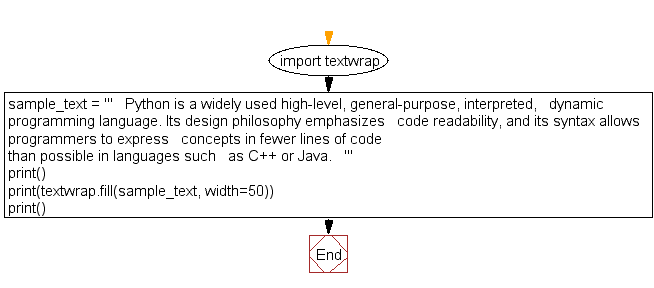﻿ Python: Display formatted text as output - w3resource

# Python: Display formatted text as output

## Python String: Exercise-26 with Solution

Write a Python program to display formatted text (width=50) as output.

Sample Solution:-

Python Code:

``````import textwrap
sample_text = '''
Python is a widely used high-level, general-purpose, interpreted,
dynamic programming language. Its design philosophy emphasizes
code readability, and its syntax allows programmers to express
concepts in fewer lines of code than possible in languages such
as C++ or Java.
'''
print()
print(textwrap.fill(sample_text, width=50))
print()
```
```

Sample Output:

``` Python is a widely used high-level, general-
purpose, interpreted,   dynamic programming
language. Its design philosophy emphasizes   code
readability, and its syntax allows programmers to
express   concepts in fewer lines of code than
possible in languages such   as C++ or Java.
```

Flowchart:Python Code Editor:

Have another way to solve this solution? Contribute your code (and comments) through Disqus.

What is the difficulty level of this exercise?

Test your Programming skills with w3resource's quiz.

﻿

## Python: Tips of the Day

Returns a list of elements that exist in both lists, after applying the provided function to each list element of both.

Example:

```def tips_intersection_by(a, b, fn):
_b = set(map(fn, b))
return [item for item in a if fn(item) in _b]

from math import floor
print(tips_intersection_by([2.1, 1.2], [2.3, 3.4],floor))
```

Output:

```[2.1]
```